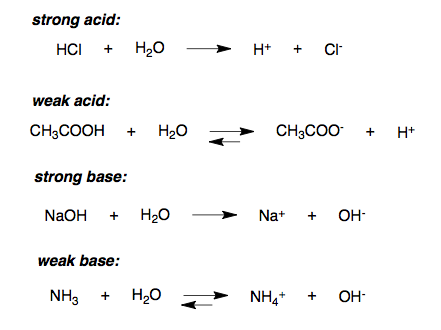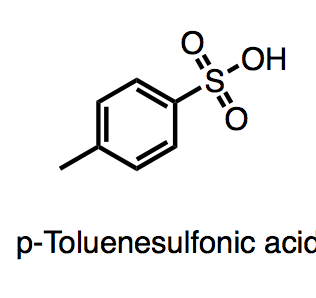## Strong Acids

#### Learning Objective

• Calculate pH for solutions of strong acids.

#### Key Points

• Strong acids can catalyze chemical reactions.
• Strong acids are defined by their pKa. The acid must be stronger in aqueous solution than a hydronium ion, so its pKa must be lower than that of a hydronium ion. Therefore, strong acids have a pKa of <-174.
• Strong acids can be organic or inorganic.
• Strong acids must be handled carefully because they can cause severe chemical burns.
• Strong acids are essential for catalyzing some reactions, including the synthesis and hydrolysis of carbonyl compounds.

#### Terms

• carbonyla divalent functional group (-CO-), characteristic of aldehydes, ketones, carboxylic acids, amides, carboxylic acid anhydrides, carbonyl halides, esters, and others.
• estera compound usually formed by condensing an alcohol and an acid and eliminating of water. it contains the functional group carbon-oxygen double bond joined via carbon to another oxygen atom
• hydrolysisa chemical process of decomposition; involves splitting a bond and adding the hydrogen cation and water’s hydroxide anion

## Definition of Strong Acids

The strength of an acid refers to the ease with which the acid loses a proton. A strong acid ionizes completely in an aqueous solution by losing one proton, according to the following equation:

$HA (aq) \rightarrow H^+ (aq) + A^-(aq)$

where HA is a protonated acid, H+ is the free acidic proton, and A is the conjugate base. Strong acids yield weak conjugate bases. For sulfuric acid, which is diprotic, the “strong acid” designation refers only to the dissociation of the first proton:

$H_2SO_4 (aq) \rightarrow H^+ (aq) + HSO_4 ^- (aq)$

More precisely, the acid must be stronger in aqueous solution than a hydronium ion (H+), so strong acids have a pKa < -1.74. An example is hydrochloric acid (HCl), whose pKa is -6.3. This generally means that in aqueous solution at standard temperature and pressure, the concentration of hydronium ions is equal to the concentration of strong acid introduced to the solution.Ionization of acids and bases in waterA strong acid ionizes completely in an aqueous solution by losing one proton (H+).

Due to the complete dissociation of strong acids in aqueous solution, the concentration of hydronium ions in the water is equal to the total concentration (ionized and un-ionized) of the acid introduced to solution:

[H+] = [A] = [HA]total and pH = −log[H+].

Strong acids, like strong bases, can cause chemical burns when exposed to living tissue.

## Examples of Strong Acids

Some common strong acids (acids with pKa < -1) include:

• Hydroiodic acid (HI): pKa = -9.3
• Hydrobromic acid (HBr): pKa = -8.7
• Perchloric acid (HClO4): pKa ≈ -8
• Hydrochloric acid (HCl): pKa = -6.3
• Sulfuric acid (H2SO4): pKa1 ≈ -3 (first dissociation only)
• p-Toluenesulfonic acid: pKa = -2.8
• Nitric acid (HNO3): pKa ≈ -1.4
• Chloric acid (HClO3): pKa ≈ 1.0p-Toluenesolfonic acidp-Toluenesulfonic acid is an example of an organic soluble strong acid, with a pKa of -2.8.

## Strong Acid Catalysis

Strong acids can accelerate the rate of certain reactions. For instance, strong acids can accelerate the synthesis and hydrolysis of carbonyl compounds. With carbonyl compounds such as esters, synthesis and hydrolysis go through a tetrahedral transition state, where the central carbon has an oxygen, an alcohol group, and the original alkyl group. Strong acids protonate the carbonyl, which makes the oxygen positively charged so that it can easily receive the double-bond electrons when the alcohol attacks the carbonyl carbon; this enables ester synthesis and hydrolysis.